In this installment, we’ll look at one of the most basic concepts of not only game theory, but of life: calculating the expected value of an event.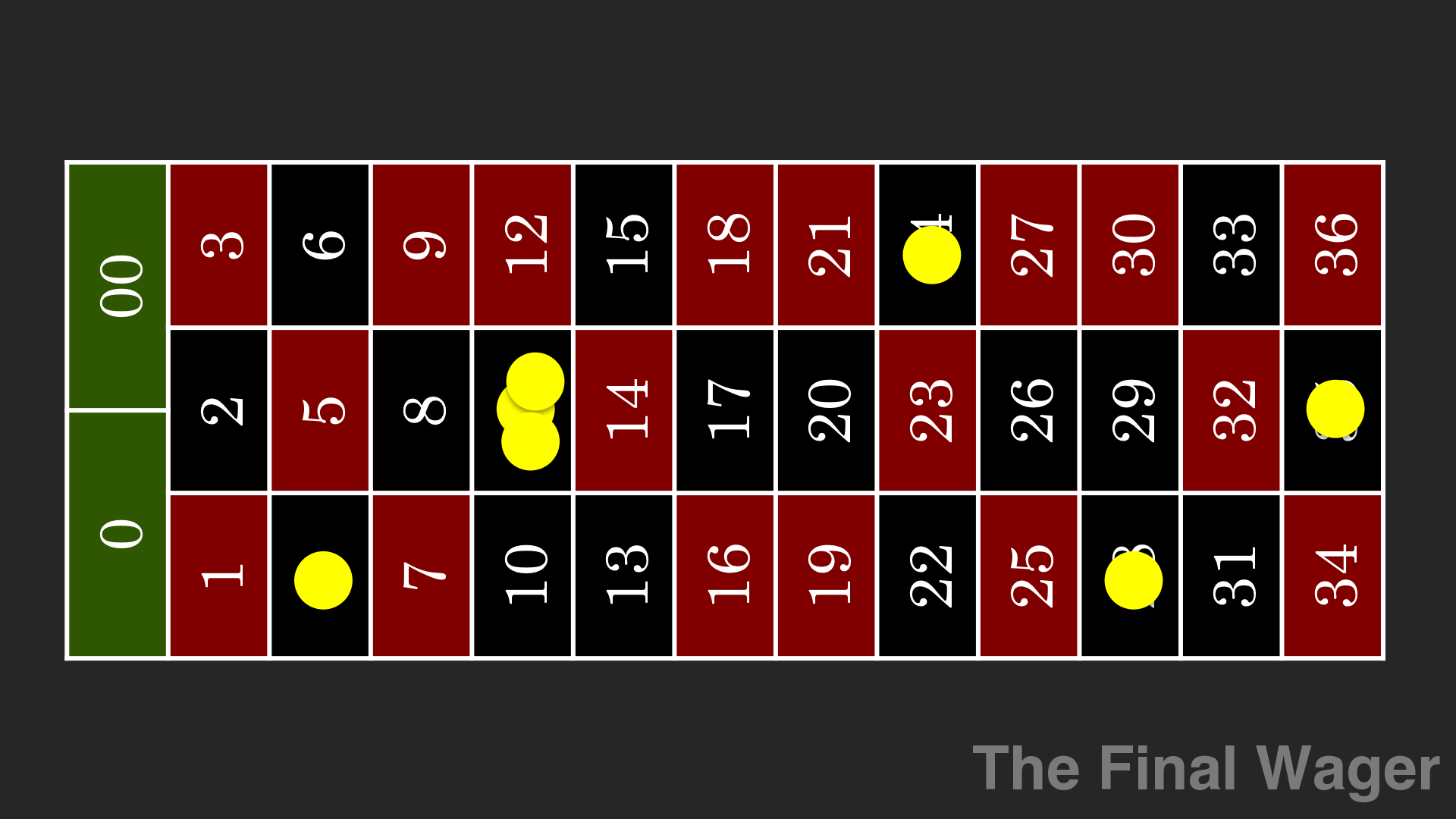Expected value is what you’d expect to average after playing a game an infinite number of times. In other words, it’s the weighted average of all outcomes, where “weighted” is when you take probability into account.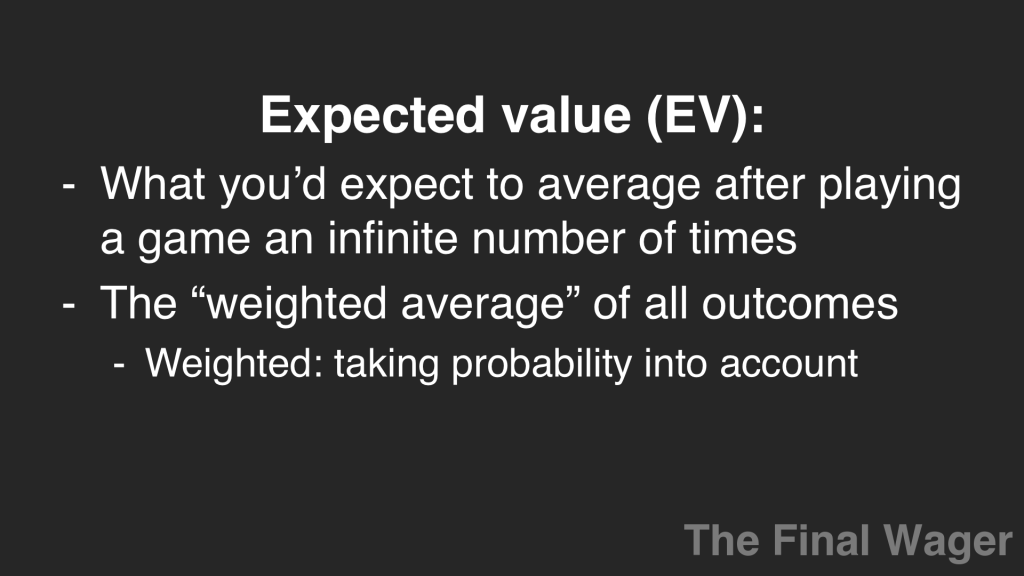In the video, I roll a die 25 times. After 25 rolls, we have an average of 2.96. Let’s see if the math bears this out.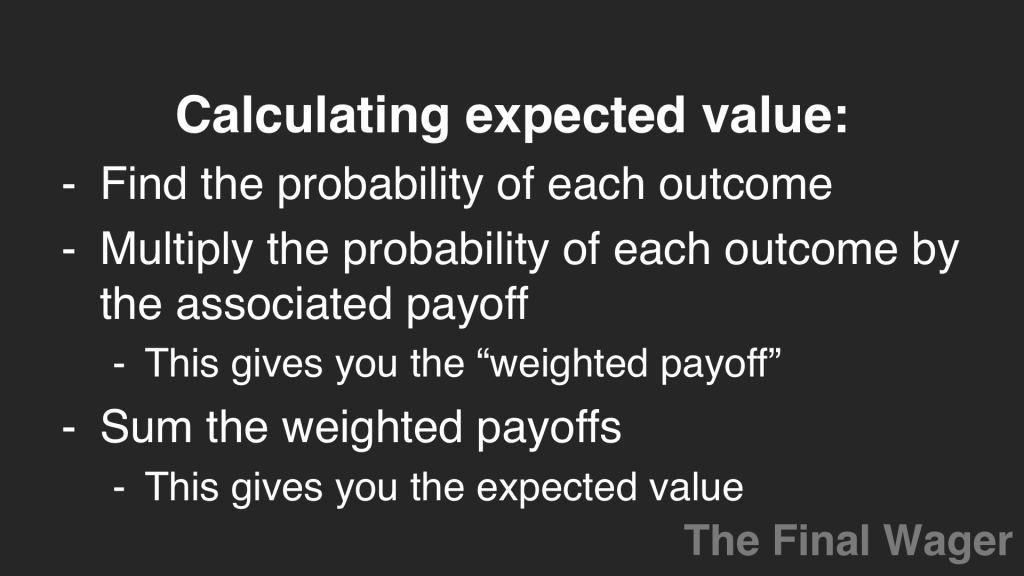To calculate the expected value, you first find the probability of each outcome. On a fair die, the odds that you roll any face is 1/6. The total of all probabilities is 1, or 100%.

Our next step in calculating expected value is to multiply the probability of each outcome by the associated payoff. This will give us the weighted payoff for that outcome. Then we add these weighted payoffs together to find our expected value: 21/6, or 3.50.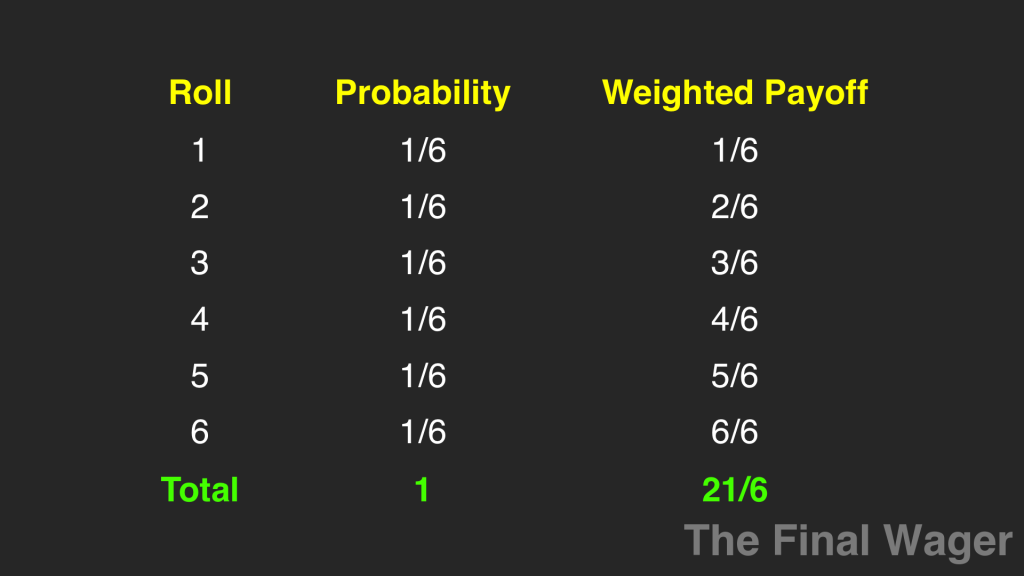Why were we so off? Well, remember that we rolled the die only 25 times. The expected value takes into account an infinite number of rolls. I rolled the die 75 more times, and after 100 rolls the average was 3.51; after 1,000 rolls (simulated – please, I have a life), the average is still right around 3.50.

Now let’s look at the payoffs associated with our mixed strategy in Final Jeopardy! wagering. We’ll need to calculate the probability of each combination: both players wager small; the leader wagers small and the trailer wagers large; the leader wagers large and the trailer wagers small; and both players wager large. If both players select make their selections randomly, they will be what are called independent events.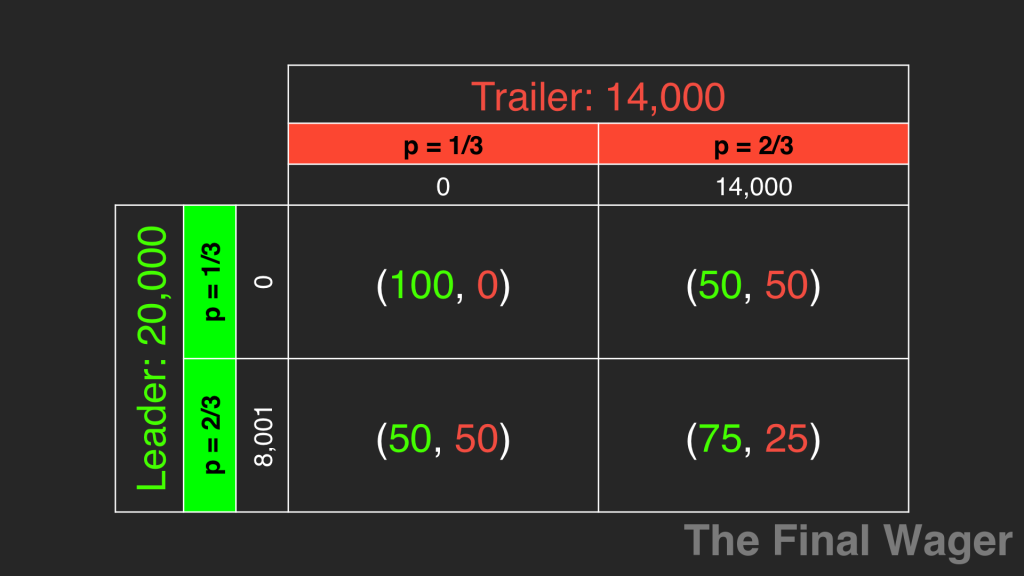Another example of an independent event is flipping a coin. Whether you flip heads on this turn will not affect the probability of flipping heads in the future. A lot of gamblers get into trouble this way, and I’ll show you why in a bit.

For independent events, we multiply the probability of each individual event occurring to figure out how often all of the events will occur. For example, what’s the probability of flipping three heads in a row?

The probability of flipping heads three times in a row, therefore, is 1/8.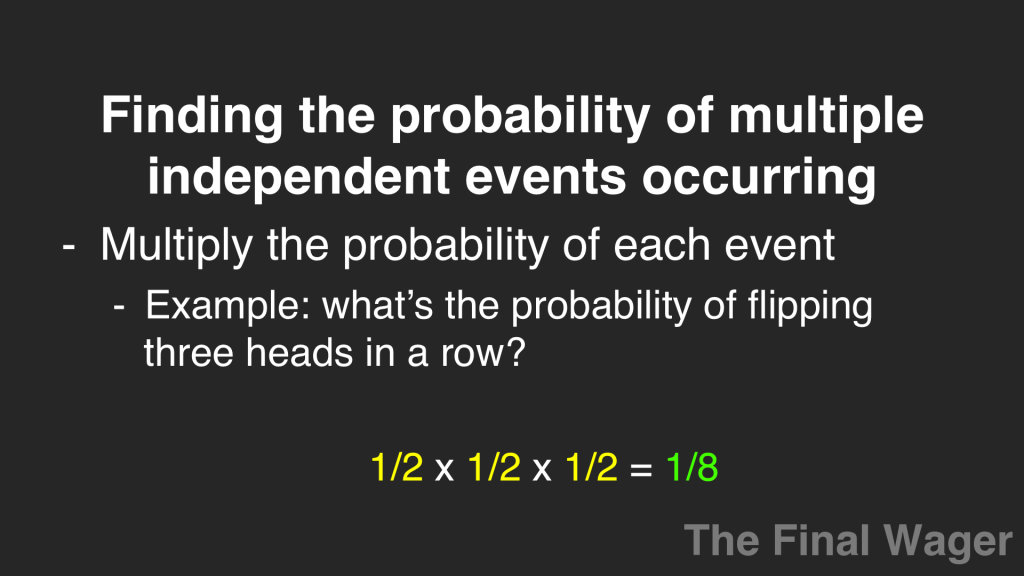Let’s return to the matrix and calculate some probabilities. If both players randomly use their optimal mixed strategies, what are the odds they’ll both wager small?

The probability that the leader wagers small is 1/3. The probability that the trailer wagers small is 1/3. 1/3 * 1/3 = 1/9. So if the players were to do this an infinite number of times, we expect to see both players wager small, on average, 1 out of every 9 times.We can do the same thing for the other cells.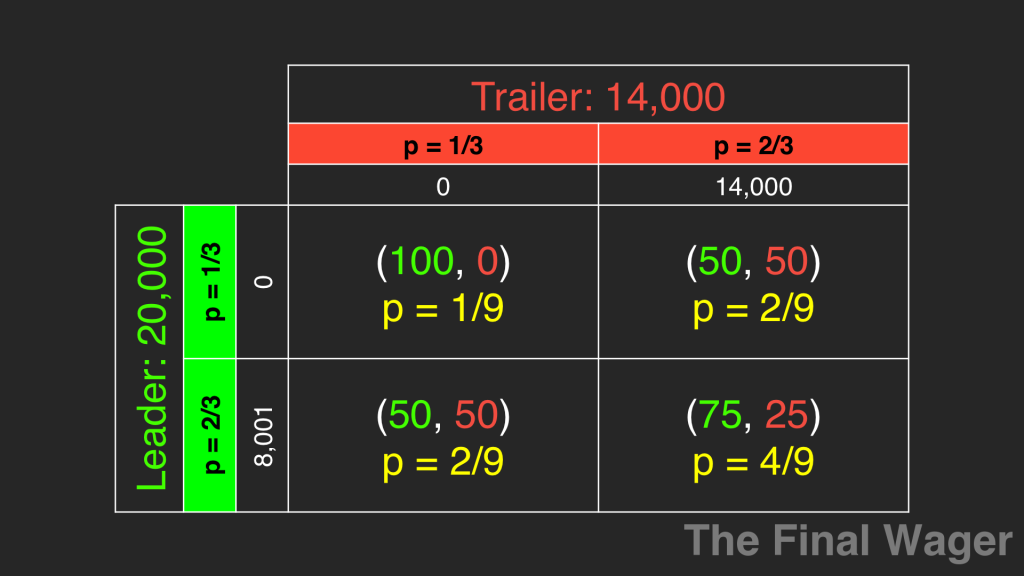Note that, here, too, adding the probabilities inside will give you a total of 1.

Step two: we multiply the probability of each event by the respective payoffs. If both players wager small, the leader will win 100% of the time, while the trailer will win 0% of the time. We multiply each of these by 1/9, the probability both will select this strategy. In that cell, we’re left with an expected payout of 100/9 for the leader, and 0 for the trailer. We do the same for the rest.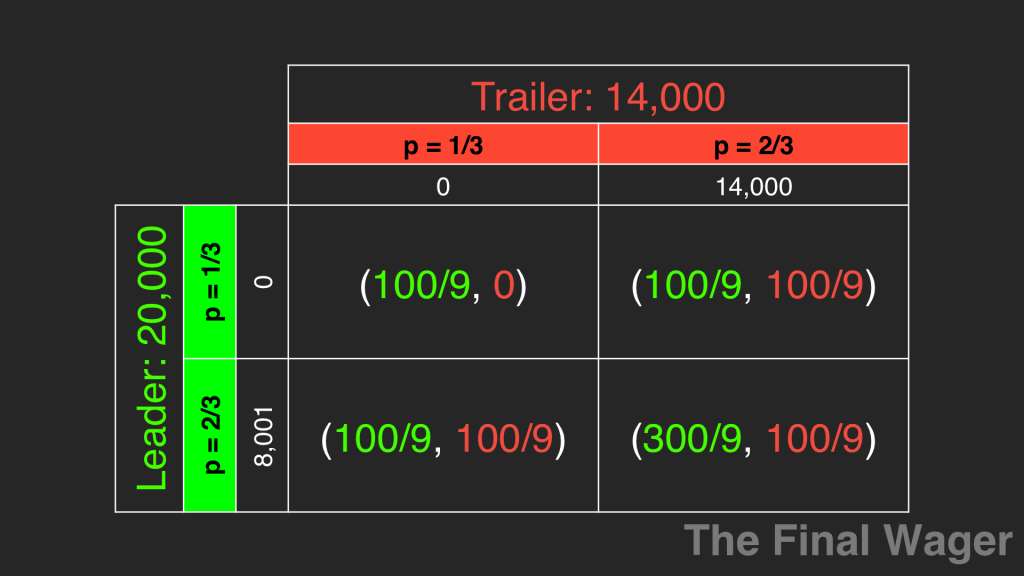Our final step is to sum the scaled payoffs. We get a grand total of 600/9 for the leader and 300/9 for the trailer. This means that when both player the optimal mixed strategy, the leader will win 2/3 of the time, and the trailer, 1/3 of the time.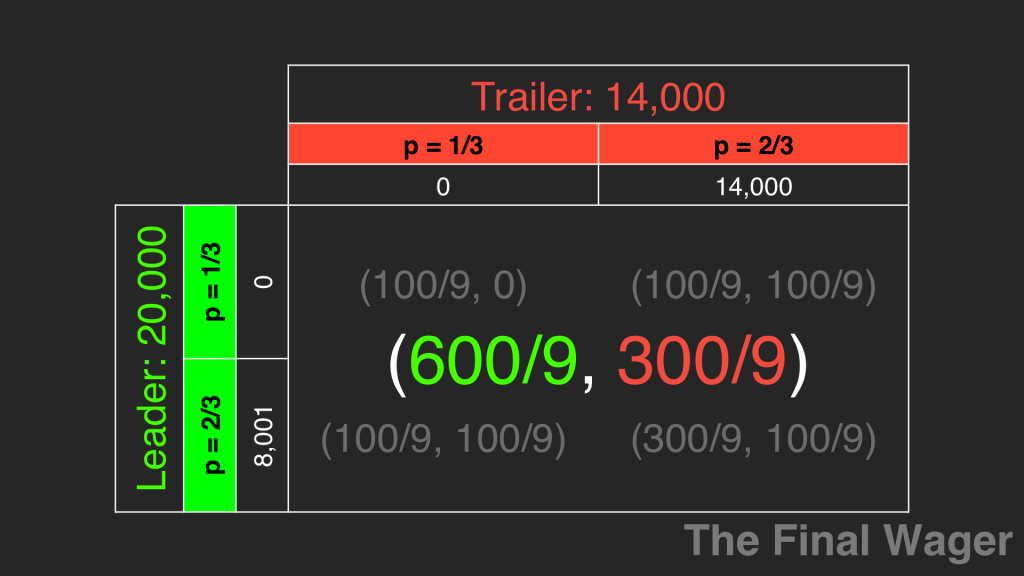Now, back to our gambling problem. Let’s say you’re playing roulette. The last seven spins have been black. Now a typical gambler might think, “RED IS DUE!” But in fact, the roulette wheel has no memory. It’s still just as likely to be black as it is to be red.This imbalance will even out over the very long term, but not necessarily on the next spin. This is called The Gambler’s Fallacy, and something to which many a poor soul has fallen victim.

And roulette isn’t even a good game to play. Craps and blackjack provide much better odds. But that’s a discussion for another time.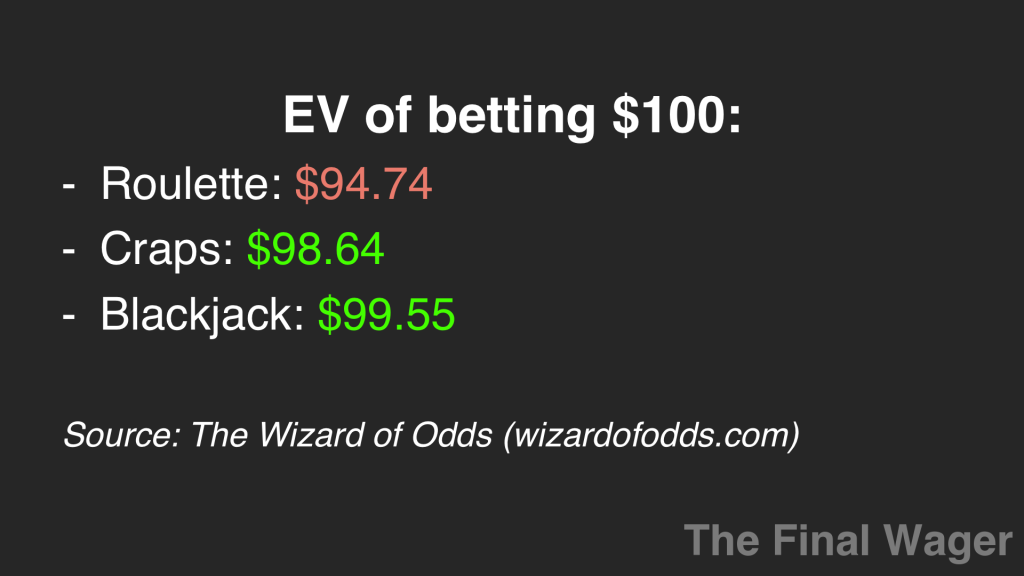SummaryTitle
The Final Wager's Guide to Game Theory - expected value, independent events, and The Gambler's Fallacy
Description
Using techniques as varied as rolling dice, flipping coins, Final Jeopardy! wagers, and roulette, 2003 Jeopardy! College Champion Keith Williams explains the concepts of expected value, independent events, and the Gambler's Fallacy.
Author
One Comment